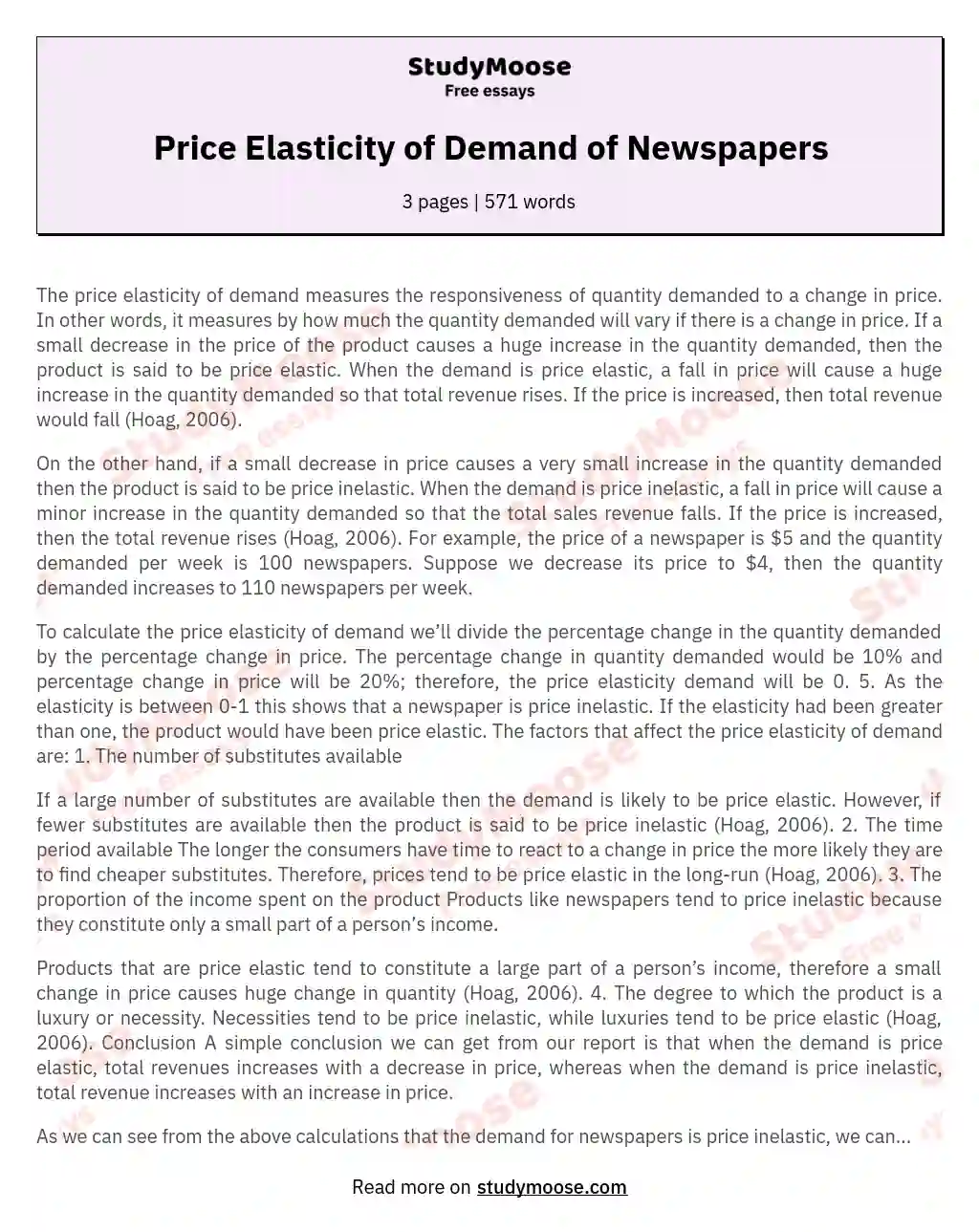# Price Elasticity of Demand of Newspapers

Categories: EconomicsPrice

The price elasticity of demand measures the responsiveness of quantity demanded to a change in price. In other words, it measures by how much the quantity demanded will vary if there is a change in price. If a small decrease in the price of the product causes a huge increase in the quantity demanded, then the product is said to be price elastic. When the demand is price elastic, a fall in price will cause a huge increase in the quantity demanded so that total revenue rises.

If the price is increased, then total revenue would fall (Hoag, 2006).

On the other hand, if a small decrease in price causes a very small increase in the quantity demanded then the product is said to be price inelastic. When the demand is price inelastic, a fall in price will cause a minor increase in the quantity demanded so that the total sales revenue falls. If the price is increased, then the total revenue rises (Hoag, 2006).

Get quality help nowMarrie pro writerVerified writer

Proficient in: Economics5 (204)

“ She followed all my directions. It was really easy to contact her and respond very fast as well. ”+84 relevant experts are online

For example, the price of a newspaper is \$5 and the quantity demanded per week is 100 newspapers. Suppose we decrease its price to \$4, then the quantity demanded increases to 110 newspapers per week.

To calculate the price elasticity of demand we’ll divide the percentage change in the quantity demanded by the percentage change in price. The percentage change in quantity demanded would be 10% and percentage change in price will be 20%; therefore, the price elasticity demand will be 0. 5. As the elasticity is between 0-1 this shows that a newspaper is price inelastic.

Get to Know The Price Estimate For Your Paper
Topic
Number of pages
Email Invalid email

You won’t be charged yet!

If the elasticity had been greater than one, the product would have been price elastic. The factors that affect the price elasticity of demand are: 1. The number of substitutes available

If a large number of substitutes are available then the demand is likely to be price elastic. However, if fewer substitutes are available then the product is said to be price inelastic (Hoag, 2006). 2. The time period available The longer the consumers have time to react to a change in price the more likely they are to find cheaper substitutes. Therefore, prices tend to be price elastic in the long-run (Hoag, 2006). 3. The proportion of the income spent on the product Products like newspapers tend to price inelastic because they constitute only a small part of a person’s income.

Products that are price elastic tend to constitute a large part of a person’s income, therefore a small change in price causes huge change in quantity (Hoag, 2006). 4. The degree to which the product is a luxury or necessity. Necessities tend to be price inelastic, while luxuries tend to be price elastic (Hoag, 2006). Conclusion A simple conclusion we can get from our report is that when the demand is price elastic, total revenues increases with a decrease in price, whereas when the demand is price inelastic, total revenue increases with an increase in price.

As we can see from the above calculations that the demand for newspapers is price inelastic, we can conclude that decreasing the price of newspapers is not a good idea as it would lead to lower revenues. I recommend that the price of the newspaper should be increased to increase revenues. Although it might slightly decrease the number of quantity demanded, most customers won’t mind the increase as newspapers make up a very small percentage of their total income. This way the revenue would increase. References Hoag, A. J. (4th Ed) (2006). Introductory Economics. World Scientific